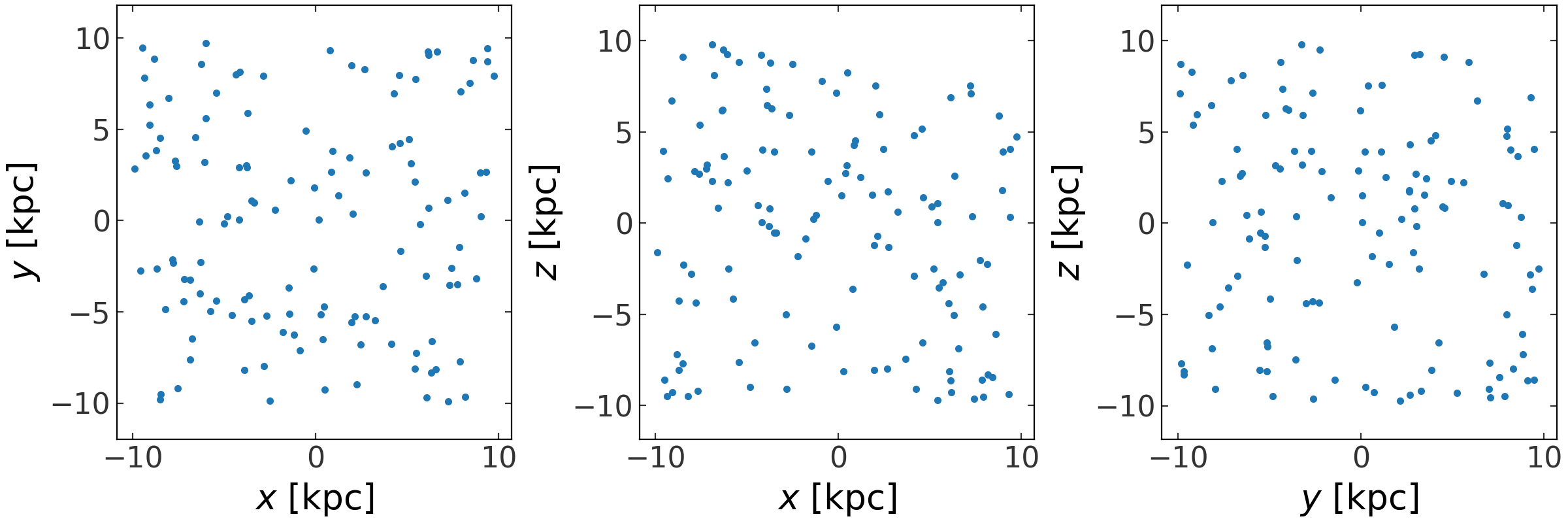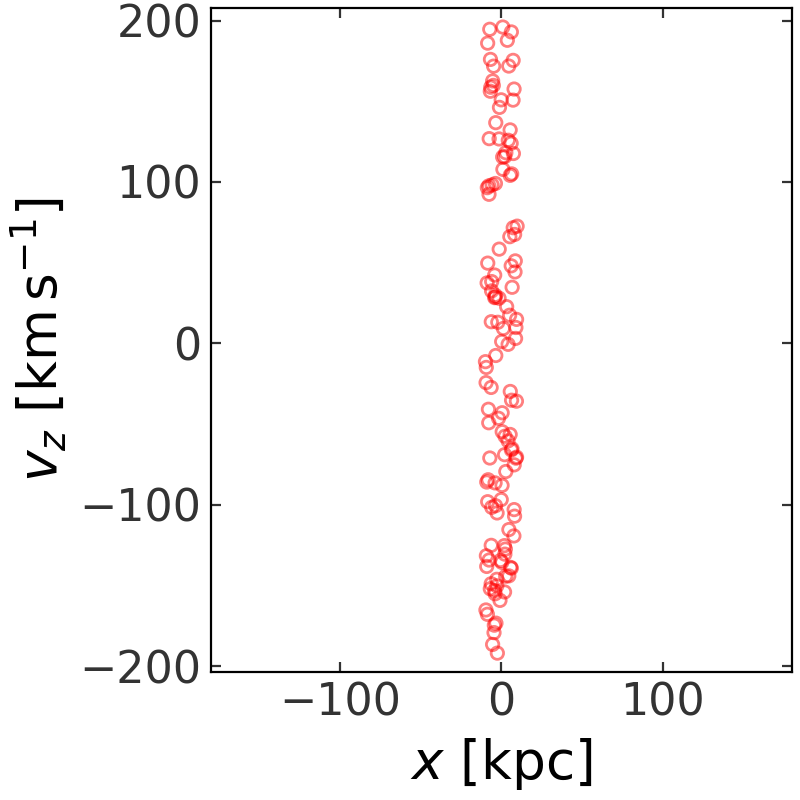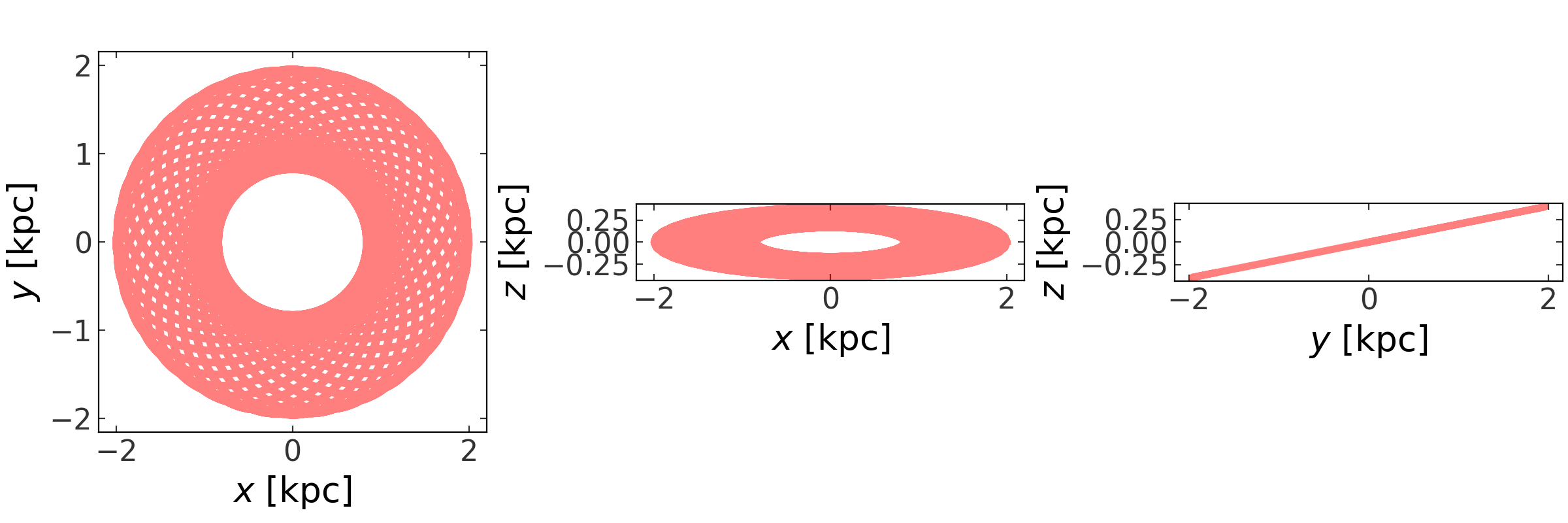# Orbit and phase-space position objects in more detail¶

We’ll assume the following imports have already been executed:

>>> import astropy.units as u
>>> import numpy as np
>>> import gala.potential as gp
>>> import gala.dynamics as gd
>>> from astropy.coordinates import (CylindricalRepresentation,
...                                  CylindricalDifferential)
>>> from gala.units import galactic
>>> np.random.seed(42)


We will also set the default Astropy Galactocentric frame parameters to the values adopted in Astropy v4.0:

>>> import astropy.coordinates as coord
>>> _ = coord.galactocentric_frame_defaults.set('v4.0')


## Introduction¶

The astropy.units subpackage is excellent for working with numbers and associated units, but dynamical quantities often contain many quantities with mixed units. An example is a position in phase-space, which may contain some quantities with length units and some quantities with velocity or momentum units. The PhaseSpacePosition and Orbit classes are designed to work with these data structures and provide a consistent API for visualizing and computing further dynamical quantities. Click these shortcuts to jump to a section below, or start reading below:

## Phase-space positions¶

The PhaseSpacePosition class provides an interface for representing full phase-space positions–coordinate positions and momenta (velocities). This class is useful as a container for initial conditions and for transforming phase-space positions to new coordinate representations or reference frames.

The easiest way to create a PhaseSpacePosition object is to pass in a pair of Quantity objects that represent the Cartesian position and velocity vectors:

>>> gd.PhaseSpacePosition(pos=[4.,8.,15.]*u.kpc,
...                       vel=[-150.,50.,15.]*u.km/u.s)
<PhaseSpacePosition cartesian, dim=3, shape=()>


By default, passing in Quantity’s are interpreted as Cartesian coordinates and velocities. This works with arrays of positions and velocities as well:

>>> x = np.arange(24).reshape(3,8)
>>> v = np.arange(24).reshape(3,8)
>>> w = gd.PhaseSpacePosition(pos=x * u.kpc,
...                           vel=v * u.km/u.s)
>>> w
<PhaseSpacePosition cartesian, dim=3, shape=(8,)>


This is interpreted as 8, 6-dimensional phase-space positions.

The class internally stores the positions and velocities as BaseRepresentation and BaseDifferential subclasses; in this case, CartesianRepresentation and CartesianDifferential:

>>> w.pos
<CartesianRepresentation (x, y, z) in kpc
[(0.,  8., 16.), (1.,  9., 17.), (2., 10., 18.), (3., 11., 19.),
(4., 12., 20.), (5., 13., 21.), (6., 14., 22.), (7., 15., 23.)]>
>>> w.vel
<CartesianDifferential (d_x, d_y, d_z) in km / s
[(0.,  8., 16.), (1.,  9., 17.), (2., 10., 18.), (3., 11., 19.),
(4., 12., 20.), (5., 13., 21.), (6., 14., 22.), (7., 15., 23.)]>


All of the components of these classes are mapped to attributes of the phase-space position class for convenience, but with more user-friendly names. These mappings are defined in the class definition of PhaseSpacePosition. For example, to access the x component of the position and the v_x component of the velocity:

>>> w.x, w.v_x
(<Quantity [0.,1.,2.,3.,4.,5.,6.,7.] kpc>, <Quantity [0.,1.,2.,3.,4.,5.,6.,7.] km / s>)


The default representation is Cartesian, but the class can also be instantiated with representation objects instead of Quantity’s – this is useful for creating PhaseSpacePosition or Orbit instances from non-Cartesian representations of the position and velocity:

>>> pos = CylindricalRepresentation(rho=np.linspace(1., 4, 4) * u.kpc,
...                                 phi=np.linspace(0, np.pi, 4) * u.rad,
...                                 z=np.linspace(-1, 1., 4) * u.kpc)
>>> vel = CylindricalDifferential(d_rho=np.linspace(100, 150, 4) * u.km/u.s,
...                               d_phi=np.linspace(-1, 1, 4) * u.rad/u.Myr,
...                               d_z=np.linspace(-15, 15., 4) * u.km/u.s)
>>> w = gd.PhaseSpacePosition(pos=pos, vel=vel)
>>> w
<PhaseSpacePosition cylindrical, dim=3, shape=(4,)>
>>> w.rho
<Quantity [1., 2., 3., 4.] kpc>


We can easily transform the full phase-space vector to new representations or coordinate frames. These transformations use the astropy.coordinates representations framework:

>>> cart = w.represent_as('cartesian')
>>> cart.x
<Quantity [ 1. ,  1. , -1.5, -4. ] kpc>
>>> sph = w.represent_as('spherical')
>>> sph.distance
<Distance [1.41421356, 2.02758751, 3.01846171, 4.12310563] kpc>


There is also support for transforming the positions and velocities (assumed to be in a Galactocentric frame) to any of the other coordinate frames. For example, to transform to Galactic coordinates:

>>> from astropy.coordinates import Galactic
>>> gal_c = w.to_coord_frame(Galactic)
>>> gal_c
<Galactic Coordinate: (l, b, distance) in (deg, deg, kpc)
[(4.40971301e-05, -6.23850462, 9.17891228),
(1.07501936e+01, -2.04017409, 9.29170644),
(2.14246214e+01,  2.65220588, 7.12026744),
(7.35169893e-05, 13.50991169, 4.23668468)]
(pm_l_cosb, pm_b, radial_velocity) in (mas / yr, mas / yr, km / s)
[( -28.11596908, -0.297625  ,    89.093095  ),
( -13.077309  ,  0.15891073,   511.60269726),
(  -7.04751509,  1.33976418, -1087.52574084),
(-206.97042166,  2.22471526,  -156.82064814)]>


We can easily plot projections of the phase-space positions using the plot method:

>>> np.random.seed(42)
>>> x = np.random.uniform(-10, 10, size=(3,128))
>>> v = np.random.uniform(-200, 200, size=(3,128))
>>> w = gd.PhaseSpacePosition(pos=x * u.kpc,
...                           vel=v * u.km/u.s)
>>> fig = w.plot()This is a thin wrapper around the plot_projections function and any keyword arguments are passed through to that function:

>>> fig = w.plot(components=['x', 'v_z'], color='r',
...              facecolor='none', marker='o', s=20, alpha=0.5)### Phase-space position API¶

#### Classes¶

 PhaseSpacePosition(pos[, vel, frame]) Represents phase-space positions, i.e.

## Orbits¶

The Orbit class inherits much of the functionality from PhaseSpacePosition (described above) and adds some additional features that are useful for time-series orbits.

An Orbit instance is initialized like the PhaseSpacePosition–with arrays of positions and velocities– but usually also requires specifying a time array as well. Also, the extra axes in these arrays hold special meaning for the Orbit class. The position and velocity arrays passed to PhaseSpacePosition can have arbitrary numbers of dimensions as long as the 0th axis specifies the dimensionality. For the Orbit class, the 0th axis remains the axis of dimensionality, but the 1st axis now is always assumed to be the time axis. For example, an input position with shape (2,128) to a PhaseSpacePosition represents 128 independent 2D positions, but to a Orbit it represents a single orbit’s positions at 128 times:

>>> t = np.linspace(0, 100, 128) * u.Myr
>>> Om = 1E-1 * u.rad / u.Myr
>>> pos = np.vstack((5*np.cos(Om*t), np.sin(Om*t))).value * u.kpc
>>> vel = np.vstack((-5*np.sin(Om*t), np.cos(Om*t))).value * u.kpc/u.Myr
>>> orbit = gd.Orbit(pos=pos, vel=vel)
>>> orbit
<Orbit ndcartesian, dim=2, shape=(128,)>


To create a single object that contains multiple orbits, the input position object should have 3 axes. The last axis (axis=2) specifies the number of orbits. So, an input position with shape (2,128,16) would represent 16, 2D orbits, each with the same 128 times:

>>> t = np.linspace(0, 100, 128) * u.Myr
>>> Om = np.random.uniform(size=16) * u.rad / u.Myr
>>> angle = Om[None] * t[:,None]
>>> pos = np.stack((5*np.cos(angle), np.sin(angle))).value * u.kpc
>>> vel = np.stack((-5*np.sin(angle), np.cos(angle))).value * u.kpc/u.Myr
>>> orbit = gd.Orbit(pos=pos, vel=vel)
>>> orbit
<Orbit ndcartesian, dim=2, shape=(128, 16)>


To make full use of the orbit functionality, you must also pass in an array with the time values and an instance of a PotentialBase subclass that represents the potential that the orbit was integrated in:

>>> pot = gp.PlummerPotential(m=1E10, b=1., units=galactic)
>>> orbit = gd.Orbit(pos=pos*u.kpc, vel=vel*u.km/u.s,
...                  t=t*u.Myr, potential=pot)


(note, in this case pos and vel were not generated from integrating an orbit in the potential pot!). However, most of the time you won’t need to create Orbit objects from scratch! They are returned from any of the numerical intergration routines provided in gala. For example, they are returned by the integrate_orbit method of potential objects and will automatically contain the time array and potential object. For example:

>>> pot = gp.PlummerPotential(m=1E10 * u.Msun, b=1. * u.kpc, units=galactic)
>>> w0 = gd.PhaseSpacePosition(pos=[10.,0,0] * u.kpc,
...                            vel=[0.,75,0] * u.km/u.s)
>>> orbit = gp.Hamiltonian(pot).integrate_orbit(w0, dt=1., n_steps=5000)
>>> orbit
<Orbit cartesian, dim=3, shape=(5001,)>
>>> orbit.t
<Quantity [0.000e+00, 1.000e+00, 2.000e+00, ..., 4.998e+03, 4.999e+03,
5.000e+03] Myr>
>>> orbit.potential


Just like for PhaseSpacePosition, we can quickly visualize an orbit using the plot method:

>>> fig = orbit.plot()Again, this is a thin wrapper around the plot_projections function and any keyword arguments are passed through to that function:

>>> fig = orbit.plot(linewidth=4., alpha=0.5, color='r')We can also quickly compute quantities like the angular momentum, and estimates for the pericenter, apocenter, eccentricity of the orbit. Estimates for the latter few get better with smaller timesteps:

>>> orbit = gp.Hamiltonian(pot).integrate_orbit(w0, dt=0.1, n_steps=100000)
>>> np.mean(orbit.angular_momentum(), axis=1)
<Quantity [0.        ,0.        ,0.76703412] kpc2 / Myr>
>>> orbit.eccentricity()
<Quantity 0.31951765618193967>
>>> orbit.pericenter()
<Quantity 10.00000005952518 kpc>
>>> orbit.apocenter()
<Quantity 19.390916871970223 kpc>


### Orbit API¶

#### Classes¶

 Orbit(pos, vel[, t, hamiltonian, potential, …]) Represents an orbit: positions and velocities (conjugate momenta) as a function of time.

#### Class Inheritance Diagram¶

Internally, both of the above classes rely on the Astropy representation transformation framework (i.e. the subclasses of BaseRepresentation and BaseDifferential). However, at present these classes only support 3D positions and differentials (velocities). The PhaseSpacePosition and Orbit classes both support arbitrary numbers of dimensions and, when relevant, rely on custom subclasses of the representation classes to handle such cases. See the N-dimensional representation classes page for more information about these classes.